##### Pre-Calculus Workbook For Dummies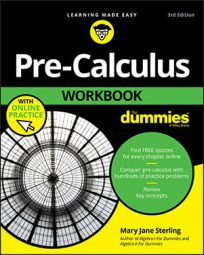Sometimes your geometry teacher may spice things up a bit with complicated polar coordinates — points with negative angles and/or radii. The following list shows you how to plot in three situations — when the angle is negative, when the radius is negative, and when both are negative.
• When the angle is negative: Negative angles move in a clockwise direction.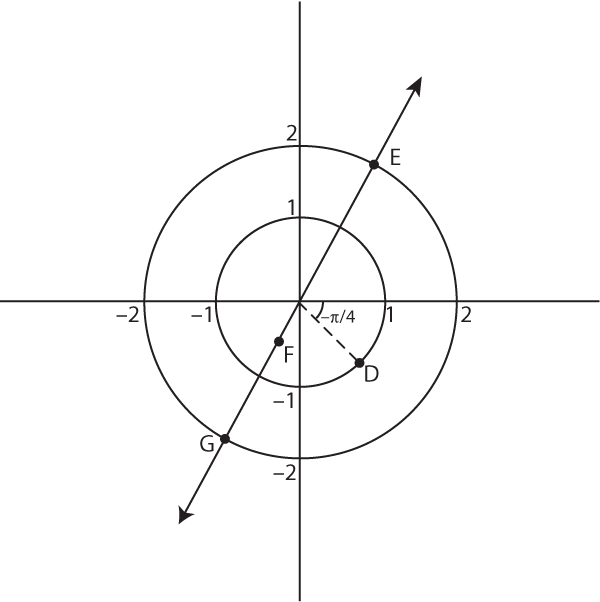Visualizing simple and complex polar coordinates

This figure shows an example point, D. To locate the polar coordinate point D at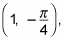first locate the angle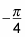and then find the location of the radius, 1, on that line.

• When the radius is negative: When graphing a polar coordinate with a negative radius, you move from the pole in the direction opposite the given positive angle (on the same line as the given angle but in the direction opposite to the angle from the pole). For example, check out point F at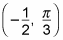in the figure.

Some teachers prefer to teach their students to move right along the x- (polar) axis for positive numbers (radii) and left for negative. Then you do the rotation for the angle in a positive direction. You’ll get to the same spot with that method.

For example, take a look at point Fin the figure. Because the radius is negative, move along the left x-axis 1/2 of a unit. Then rotate the angle in the positive direction (counterclockwise) pi/3 radians. You should arrive at your destination, point F.

• When both the angle and radius are negative: To express a polar coordinate with a negative radius and a negative angle, locate the terminal side of the negative angle first and then move in the opposite direction to locate the radius. For example, point G in the figure has these characteristics at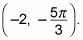Indeed, except the origin, each given point can have the following four types of representations:

For example, point E in the figure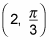can have three other polar coordinate representations with different combinations of signs for the radius and angle: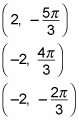When polar graphing, you can change the coordinate of any point you’re given into polar coordinates that are easy to deal with (such as positive radius, positive angle).